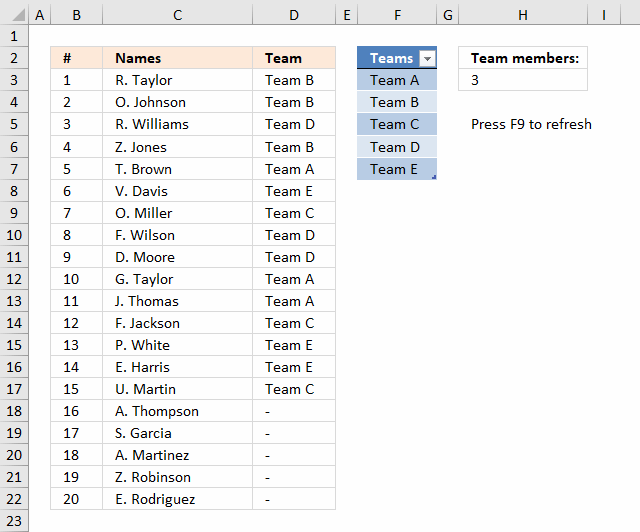Author: Oscar Cronquist Article last updated on January 04, 20191 - I see you could change the formula to have the experssion COUNTIF(\$C\$1:C1, \$E\$2:\$E\$5)<5 changed so the maximum number of team members looks to a cell (say E9) so the number of people per team could be easily changed eg COUNTIF(\$C\$1:C1, \$E\$2:\$E\$5)<E9

2 - In (virtual) sailing team racing, competition is usually 3 vs 3, but could be adjusted to a 4 vs 4, 3 vs 2 or 4 vs 3 depending on the number of skippers available to race. Could the example be modified to assign a skipper to a team given these optimum arrangements? (eg for say 12 skippers entered it would do 2 sets of 3 vs 3, for 8 skippers it would do one 4 vs 4, etc)

The image above demonstrates a worksheet containing Team names in an Excel defined table cell range F3:F7, you don't need to adjust cell references in the formula if you add or delete team names. It is dynamic and updates instantly.

Enter the number of members in each team in cell H3, the formula refreshes automatically. It randomly assigns teams to players, press F9 to refresh the formula output.

Array formula in cell D3:

=IFERROR(INDEX(Table1[Teams], LARGE((COUNTIF(\$D\$2:D2, Table1[Teams])<\$H\$3)*(MATCH(ROW(Table1[Teams]),ROW(Table1[Teams]))), RANDBETWEEN(1, SUMPRODUCT(--(COUNTIF(\$D\$2:D2, Table1[Teams])<\$H\$3))))), "-")

To enter an array formula, type the formula in a cell then press and hold CTRL + SHIFT simultaneously, now press Enter once. Release all keys.

The formula bar now shows the formula with a beginning and ending curly bracket telling you that you entered the formula successfully. Don't enter the curly brackets yourself.

Copy cell D3 and paste it down as far as necessary.

### Explaining formula in cell D3

#### Step 1 - Count previous values

The COUNTIF function counts values based on a condition or criteria, the first argument contains an expanding cell reference, it grows when the cell is copied to cells below. This makes the formula aware of values displayed in cells above. 0 (zero) indicates values that not yet have been displayed.

COUNTIF(\$D\$2:D2, Table1[Teams])<\$H\$3

becomes

COUNTIF("Team", {"Team A"; "Team B"; "Team C"; "Team D"; "Team E"})<\$H\$3

becomes

{0;0;0;0;0}<3

and returns

{TRUE; TRUE; TRUE; TRUE; TRUE}

#### Step 2 - Create row numbers

This step is needed to create a sequence of numbers that will be used to get the correct value in a later step.

MATCH(ROW(Table1[Teams]),ROW(Table1[Teams]))

becomes

MATCH(ROW({"Team A"; "Team B"; "Team C"; "Team D"; "Team E"}),ROW({"Team A"; "Team B"; "Team C"; "Team D"; "Team E"}))

becomes

MATCH({3;4;5;6;7}, {3;4;5;6;7})

and returns {1;2;3;4;5}.

#### Step 3 - Multiply arrays

(COUNTIF(\$D\$2:D2, Table1[Teams])<\$H\$3)*(MATCH(ROW(Table1[Teams]),ROW(Table1[Teams])))

becomes

{TRUE; TRUE; TRUE; TRUE; TRUE}* {1;2;3;4;5}

and returns {1;2;3;4;5}.

#### Step 4 - Extract random row number

The LARGE function returns the k-th largest number, LARGE( array , k). The second argument k is a random number from 1 to n.

LARGE((COUNTIF(\$D\$2:D2, Table1[Teams])<\$H\$3)*(MATCH(ROW(Table1[Teams]),ROW(Table1[Teams]))), RANDBETWEEN(1, SUMPRODUCT(--(COUNTIF(\$D\$2:D2, Table1[Teams])<\$H\$3))))

becomes

LARGE({1;2;3;4;5}, RANDBETWEEN(1, SUMPRODUCT(--(COUNTIF(\$D\$2:D2, Table1[Teams])<\$H\$3))))

becomes

LARGE({1;2;3;4;5}, RANDBETWEEN(1, SUMPRODUCT({1; 1; 1; 1; 1})))

becomes

LARGE({1;2;3;4;5}, RANDBETWEEN(1, 5))

becomes

LARGE({1;2;3;4;5}, 2)

and returns 2. This is a random number between 1 and 5.

#### Step 5 - Return value

The INDEX function returns a value based on a cell reference and column/row numbers.

INDEX(Table1[Teams], LARGE((COUNTIF(\$D\$2:D2, Table1[Teams])<\$H\$3)*(MATCH(ROW(Table1[Teams]),ROW(Table1[Teams]))), RANDBETWEEN(1, SUMPRODUCT(--(COUNTIF(\$D\$2:D2, Table1[Teams])<\$H\$3)))))

becomes

INDEX(Table1[Teams], 2)

and returns "Team B" in cell D3.

#### Step 6 - Trap errors

The formula returns errors when all teams are populated, the IFERROR function converts the errors into a given value, in this case "-".

Note: The IFERROR function catches all kinds of errors in your formula, this might cause you to not detect other errors in your formula. Use this function with caution.

IFERROR(INDEX(Table1[Teams], LARGE((COUNTIF(\$D\$2:D2, Table1[Teams])<\$H\$3)*(MATCH(ROW(Table1[Teams]),ROW(Table1[Teams]))), RANDBETWEEN(1, SUMPRODUCT(--(COUNTIF(\$D\$2:D2, Table1[Teams])<\$H\$3))))), "-")

### Get the Excel fileDynamic-team-generator.xlsx